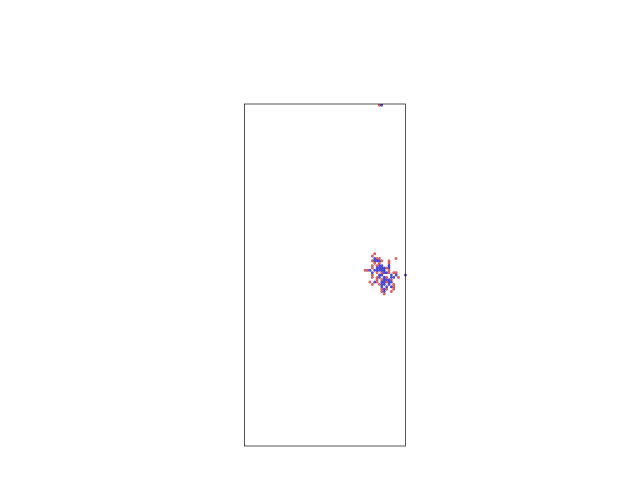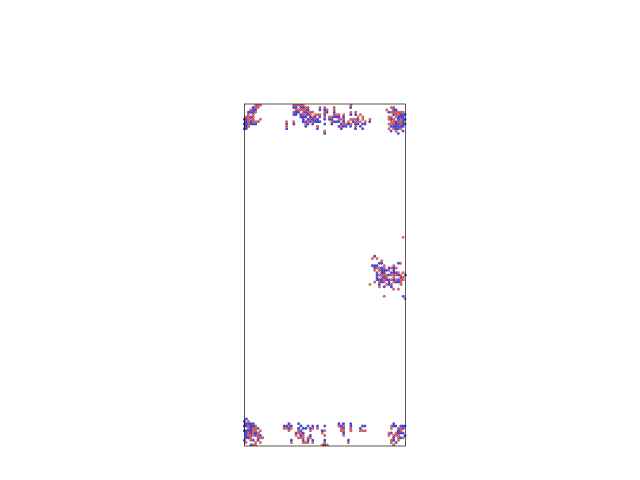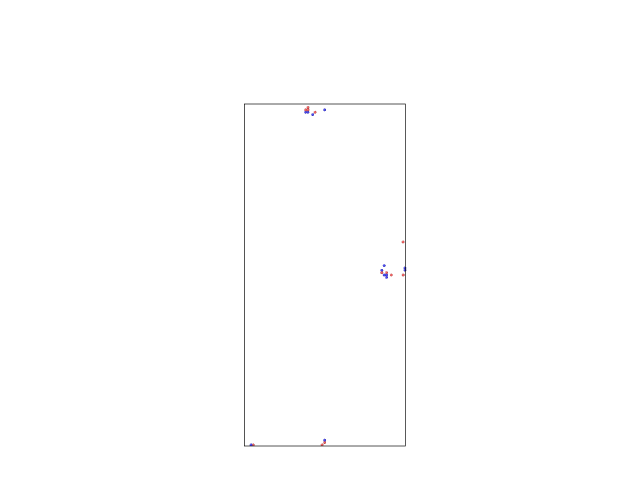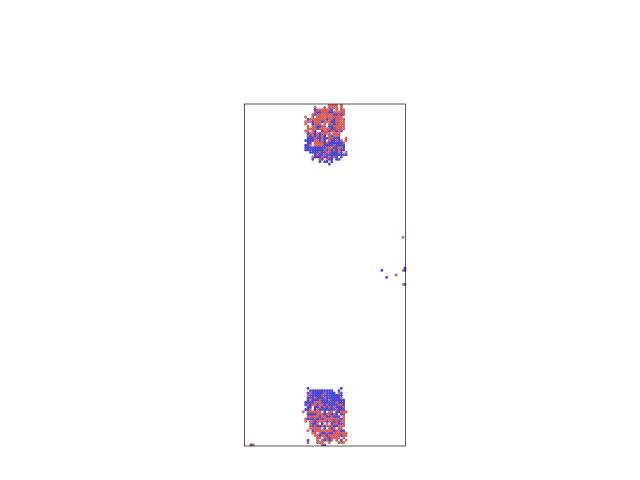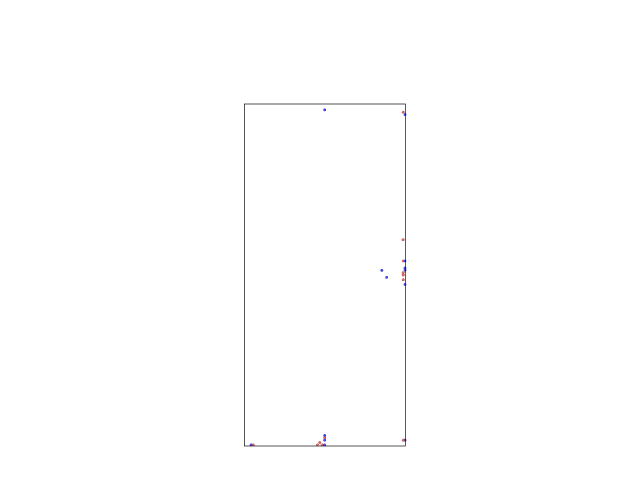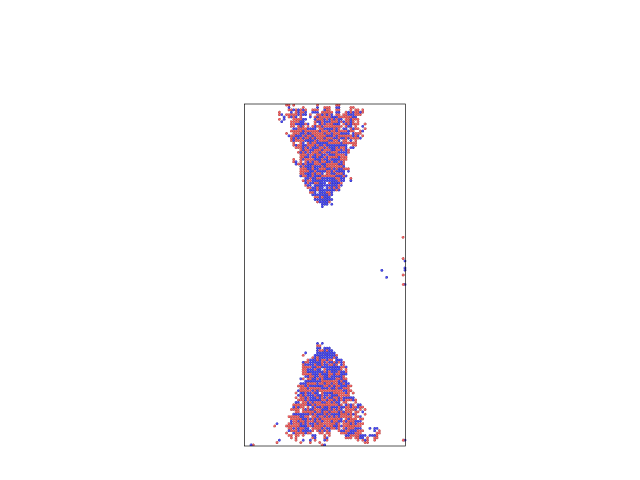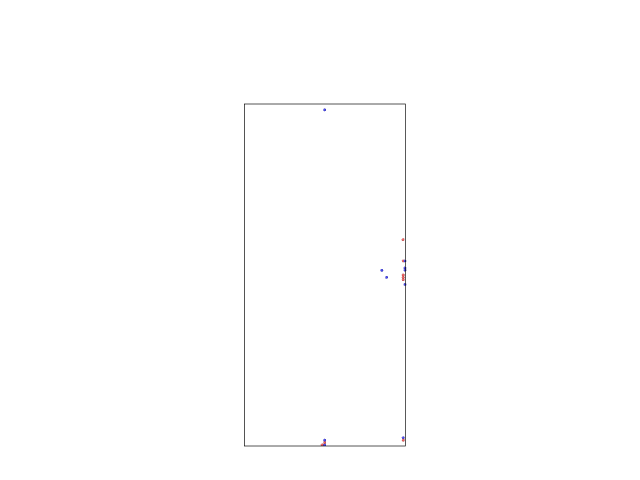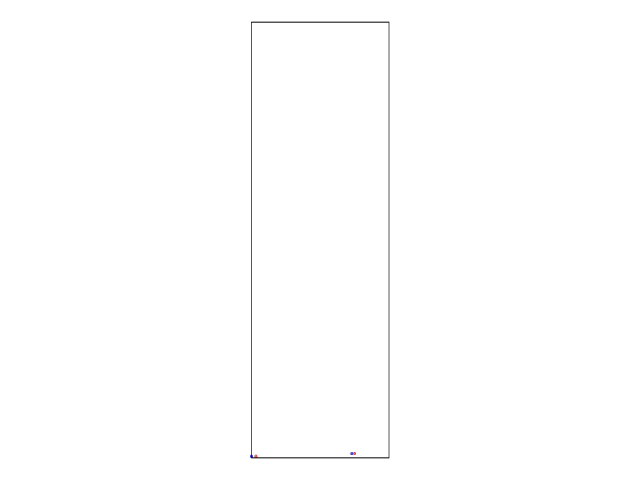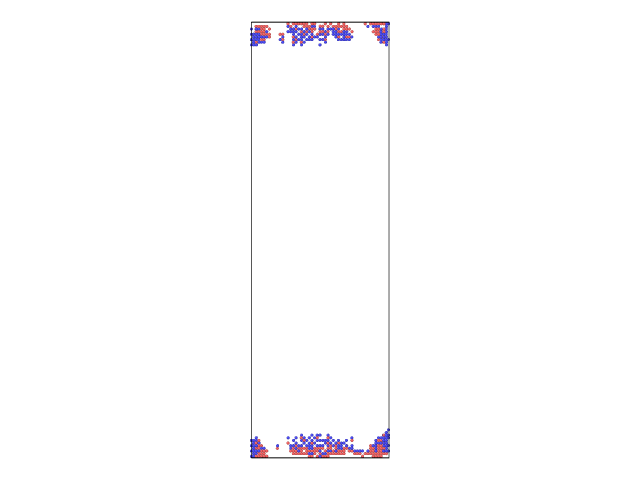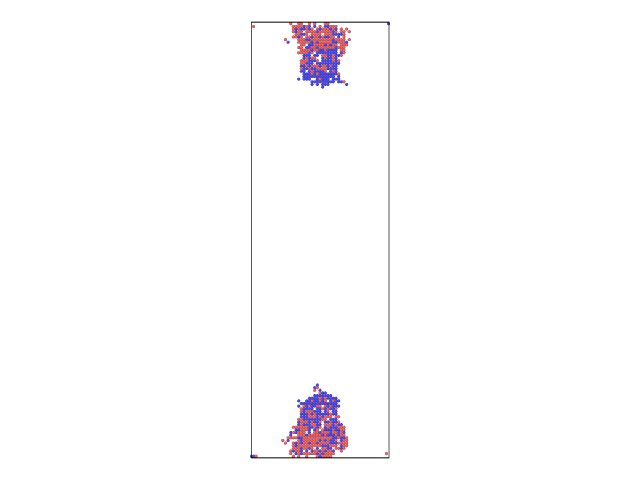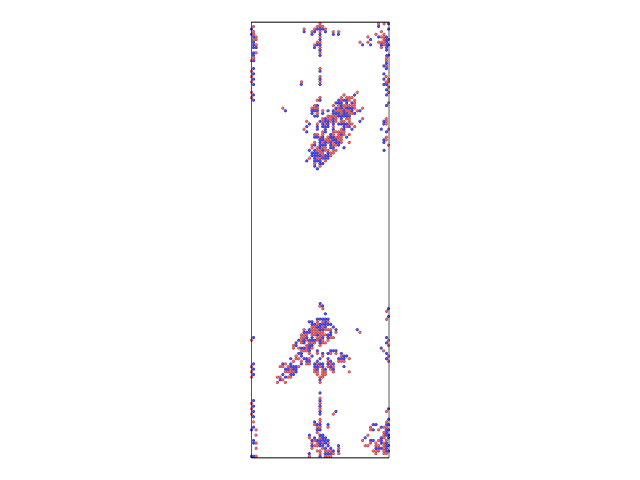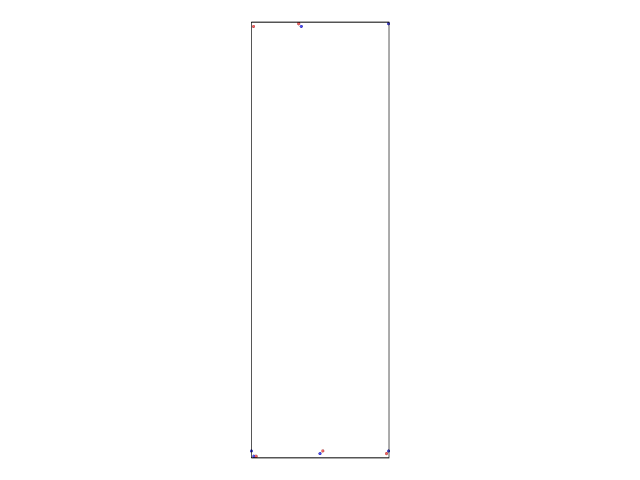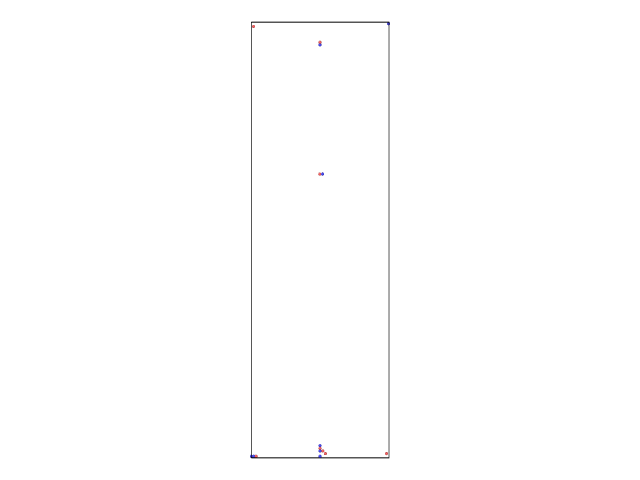# Unexpected defects generated during simulation of metal nanowire, how can I solve this problem?

Dear everyone,
I am trying to run cascade simulations of metal cylinder, but when I increased the radius of my cylinder, some unexpected defects shown at the ends. Here are the details.
The boundary conditions are s s p. And a cylinder was constructed using ‘region rwire cylinder z 0 0 \$r 0 l’ and the length l equals r\*4\+4, in lattice units\. Then I used ‘min\_style cg’ and ‘fix npt\_relax wire npt temp 300 300 0\.1 z 0 0 1 drag 0\.2’ to thermally relax the wire\. After relaxation, a restart file was written\. Then I load the restart file by ‘read\_restart’ command\. After that the following command is used\. change\_box all x final (-v_r) \(v\_r\) y final (-v_r) \$(v_r) z final 0 l boundary p p p region PKA\_Au sphere 0 (-v_r) \$(v_l/2) 0.1 side in
delete_atoms region PKA_Au compress no
create_atoms 1 region PKA_Au
change_box all boundary s s p
The PKA is supposed to be created at the surface or the center of the cylinder. Three regions were then defined by ‘region rinterior cylinder z 0 0 r 2 (v_l-2), region rexterior1 cylinder z 0 0 \$r 0 2, region rexterior2 cylinder z 0 0 r (v_l-2) \$l’. The two exterior regions are 2 atoms layers at the top and bottom of my cylinder, respectively. At last, the fixes which are usually used in cascade simulation is applied, which are ‘fix boundary1 exterior1 temp/rescale 1 \$T \$T 0.5 1.0, fix boundary2 exterior2 temp/rescale 1 \$T T 0\.5 1\.0, fix NVE all nve, fix recom all recenter INIT INIT INIT units box’\. My simulations seem got reasonable results when the scripts were applied to cylinders of radius r=10&amp;length l=r\*4\+4=44 and r=8&amp;l=32\+4=36\. Unexpected results happened when the radius was increased to r=13 and above, some interstitial sites and vacancy sites will appear at the top and/or bottom of the cylinder\. One example is the pictures in the attachments which show the case of r=17&amp;l=72\. I also tried to run the simulation in which PKA is induced but with ZERO velocity, which means only one difference is that command ‘velocity Cascade\_Au set 0\.000000 312\.732627 0\.000000 units box’ in my second input file is commented\. I got similar results in this situation\. And the pictures are also shown in the attachment as an example of r=13&amp;l=6\*r\+4=82\. As can be seen, by applying W\-S analysis, interstitials and vacancies are appeared in the cylinder\. I made several attempts with different radii and length radius ratios\. It seems these unexpected defects are more easily shown in cylinder with larger radius and larger length\-radius ratio\. When r=8, these defects show at the ends until l=8\*10\+4=84 \(ratio 8\), with limited values\. But with r=13, it shows when l=4\*13\+4=56 \(ratio 4\), with much more defects\. One odd thing to me is, if I further comment all the following lines, \#change\_box all x final (-v_r) \(v\_r\) y final (-v_r) \$(v_r) z final 0 l boundary p p p \#region PKA\_Au sphere 0 (-v_r) \$(v_l/2) 0.1 side in
#delete_atoms region PKA_Au compress no
#create_atoms 1 region PKA_Au
#change_box all boundary s s p
The defects will NOT appear in my nanowires of r=13&l=6*13+4=82 and r=17&l=17*4+4=72.
In short words, if I introduce PKA with energy 1keV or zero velocity, the unexpected defects will show at the ends of the cylinders. The attachments show the cases of two sizes, r=13&l=82 and r1=17&l=72. By the way, in most cases, the defects will eventually disappear at the end of my simulation which lasts about 80ps. But, if I comment the lines between the two 'change_box' commands, nearly no defects show in these two nanowires.
Though I tried a lot, due to the limited experience and knowledge, I cannot solve my problem. Could anyone tell me the reason? Or please tell me how to fix this problem.

With My Best Regards
Liu